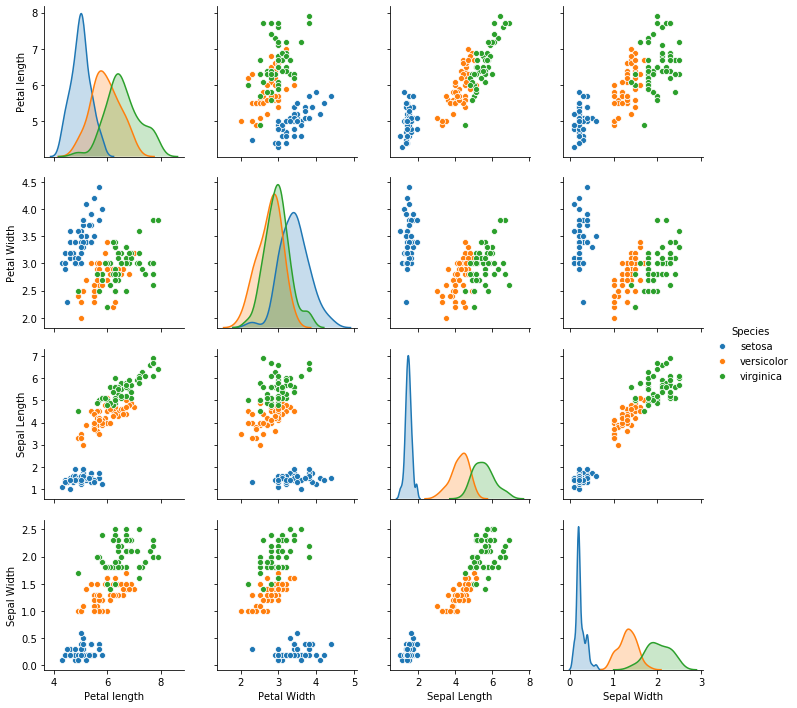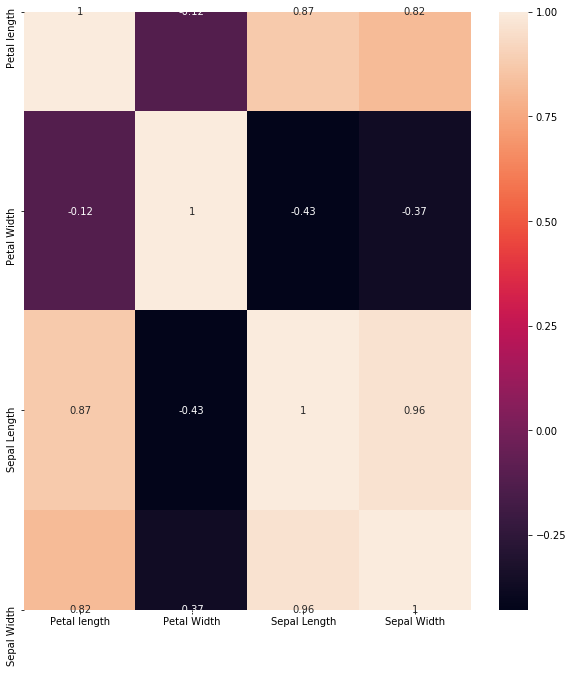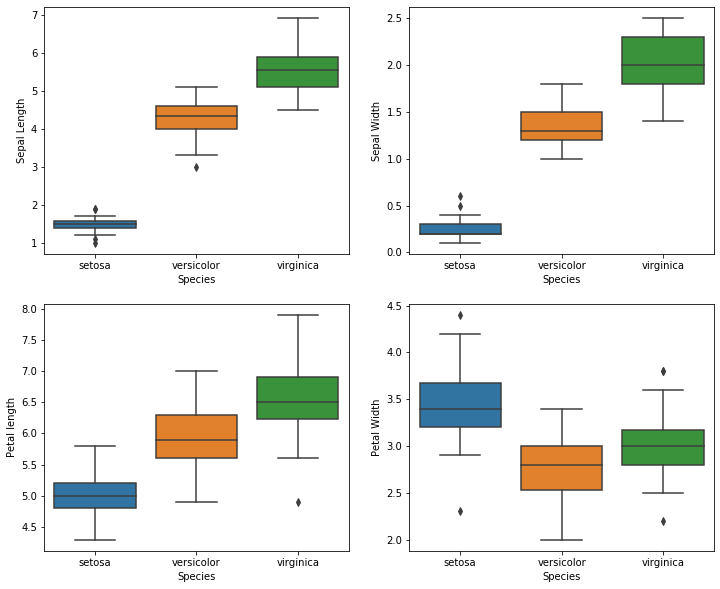top of page
Search

# Unsupervised Machine Learning: Classification of Iris data set

The Iris data set is the 'Hello world' in the field of data science.This data sets consists of 3 different types of irises’ (Setosa, Versicolour, and Virginica) petal and sepal length, stored in a 150x4 numpy.ndarray.

The rows being the samples and the columns being: Sepal Length, Sepal Width, Petal Length and Petal Width.

The data set is often used in data mining, classification and clustering examples and to test algorithms.

First we will load the Iris data set from sklearn.datasets and then we will convert it into a dataframe using pandas so that we can easily work with it. We will use matplotlib and seaborn to visualise the data.

```from sklearn.datasets import load_iris
import matplotlib.pyplot as plt
import pandas as pd
import numpy as np
import seaborn as sns

data=pd.DataFrame(dataset['data'],columns=['Petal length','Petal Width','Sepal Length','Sepal Width'])
data['Species']=dataset['target']
data['Species']=data['Species'].apply(lambda x: dataset['target_names'][x])Now, we will explore the data to understand it better and to make it suitable to be fed to the machine learning algorithm.

We will check for missing values in the data:

`data.isnull().sum()`We see that there are no missing value in this data set.

We will check the information about our DataFrame including the index dtype and columns, non-null values and memory usage, by callling the info( ) function.

`data.info()`We can see that there are 150 total rows and there is no missing value. The type of each column is also specified.

Now, we will look at the descriptive statistics of the data using the describe( ) function.

`data.describe()`We will now make a pairplot using seaborn to visualize the relationship between the columns of the data frame for different species.

`sns.pairplot(data,hue='Species')`We can see the range of petal length, petal width, sepal length and sepal width for the 3 species easily from the graph. Thus, we can determine to which species a new data point might belong to.

We can also visualise our dataframe using a heatmap,a violin plot and a boxplot; as follows:

• Heatmap:

```plt.figure(figsize=(10,11))
sns.heatmap(data.corr(),annot=True)
plt.plot()```• Violin plot:

```plt.figure(figsize=(12,10))
plt.subplot(2,2,1)
sns.violinplot(x='Species',y='Sepal Length',data=data)
plt.subplot(2,2,2)
sns.violinplot(x='Species',y='Sepal Width',data=data)
plt.subplot(2,2,3)
sns.violinplot(x='Species',y='Petal length',data=data)
plt.subplot(2,2,4)
sns.violinplot(x='Species',y='Petal Width',data=data)```• Boxplot:

```plt.figure(figsize=(12,10))
plt.subplot(2,2,1)
sns.boxplot(x='Species',y='Sepal Length',data=data)
plt.subplot(2,2,2)
sns.boxplot(x='Species',y='Sepal Width',data=data)
plt.subplot(2,2,3)
sns.boxplot(x='Species',y='Petal length',data=data)
plt.subplot(2,2,4)
sns.boxplot(x='Species',y='Petal Width',data=data)```Now, we need to replace the values of species column with some numerical value so that it will be easier for the machine to understand. This process is called encoding. We will replace 'setosa' with '0', 'versicolor' with '1' and 'virginica' with '2'.

```from sklearn import preprocessing
le = preprocessing.LabelEncoder()
le.fit(data['Species'])
y =le.transform(data['Species'])
y```We can see that the species column have been encoded using LabelEncoder from preprocessing class of sklearn library.

Now, our data is ready to be trained on different classification models.

Here we will be training our data on DecisionTreeClassifier, SupportVectorClassifier, RandomForestClassifier and KNeighboursClassifiers, then we will determine which algorithm worked better.

• DecisionTreeClassifier

```from sklearn.tree import DecisionTreeClassifier
from sklearn.model_selection import cross_val_score

DT = DecisionTreeClassifier(random_state=0)
score1=cross_val_score(DT, data[['Petal length','Petal Width','Sepal Length']], y, cv=10)
print(score1.mean())```

Output: 0.9466666666666667

• SupportVectorClassifier

```from sklearn.svm import SVC

svm_clf =SVC(gamma='auto')

score2=cross_val_score(svm_clf, data[['Petal length','Petal Width','Sepal Length']], y, cv=10)
print(score2.mean())```

Output: 0.9533333333333334

• RandomForestclassifier

```from sklearn.ensemble import RandomForestClassifier

RFC = RandomForestClassifier(max_depth=2, random_state=0)
score3=cross_val_score(RFC, data[['Petal length','Petal Width','Sepal Length']], y, cv=10)
print(score3.mean())```

Output: 0.9133333333333333

• KNeighboursClassifiers

```from sklearn.neighbors import KNeighborsClassifier

KNN = KNeighborsClassifier(n_neighbors=3)
score4=cross_val_score(RFC, data[['Petal length','Petal Width','Sepal Length']], y, cv=10)
print(score4.mean())```

Output: 0.9133333333333333

The output of the above code snippets shows the accuracy of the model. The higher the accuracy the better the model at classifying correctly. We can see that SupportVectorClassifier was the best at classifying correctly followed by DecisionTreeClassifier and then by RandomForestClassifier and KNeighboursClassifiers both of which performed equally.

We can't say that this is always true. Different algorithms work better in different situations. So we should always train our data on a number of algorithms and we should then select the best.